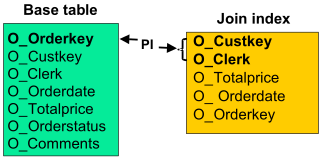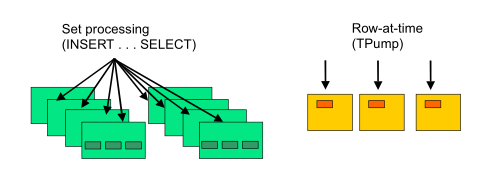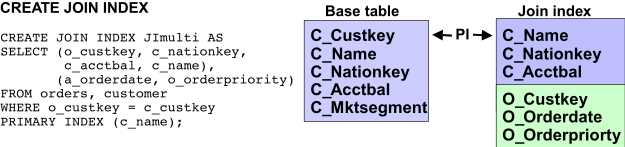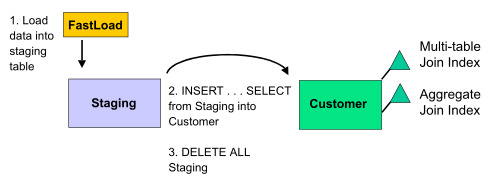16.10 - Join Indexes and Tactical Queries - Teradata Database

Product
Release Number
16.10
Release Date
June 2017
Content Type
User Guide
Publication ID
B035-1094-161K
Language
English (United States)

Single-Table Join Indexes

One of the most useful constructs for tactical queries is the single-table join index. Because you can define a primary index for the join index composed of different columns than those used to define the base table primary index, you can create an alternative method of directly accessing data in the associated base table.

For example, you could create a join index on the orders table that includes only a subset of the columns that a particular tactical query application might require. In the example shown in the following graphic, assume that the application has available a value for o_custkey and the clerk that placed the order, but does not have a value for o_orderkey. Specifying the primary index defined for the join index OrderJI supports direct access to order base table using the single-table join index OrderJI.

CREATE JOIN INDEX OrderJI AS
SELECT o_custkey, o_clerk, o_totalprice, o_orderdate, o_orderkey
FROM orders
PRIMARY INDEX(o_custkey,o_clerk);A test against an orders table with 75 million rows, both with and without the OrderJI join index, returned the following response times from the query and join index above:

If the query is run … The response time is …
without the join index 1:55.
with the join index subsecond.

The larger the base table is, the longer it takes to process via a table scan, and the greater the benefit a join index providing single-AMP access provides. In this example, the join index took 8:23 to create.

A single-table join index like this one is particularly useful when the tactical application does not have the primary index of the base table available, but has an alternative row identifier. This might be the case when a social security number is available, but a member ID, the primary index of the base table, is not. Single-AMP access would still be achievable using the join index if its primary index is defined on social security number.

Aggregate Join Indexes

If your application supports repeated access to the same table using aggregation along the same set of dimensions, you should consider using aggregate join indexes to enhance the performance of those queries.

If you include one or more aggregating columns in the join index select list, then that index is an aggregate join index. Aggregate join indexes are dynamic summary tables, not snapshots. For example, the following aggregate join index computes a running sum on o_totalprice from the orders table:

CREATE JOIN INDEX ordersum AS
SELECT o_clerk, SUM(o_totalprice) AS sumprice
FROM orders
GROUP BY o_clerk
PRIMARY INDEX(o_clerk);

Each time the o_totalprice column of orders is updated, a new sum is computed and stored in the ordersum aggregate join index. If there are frequent queries throughout the day that request summaries of the total prices for orders placed by a specific clerk, this join index would be able to deliver response times suitable for tactical queries like the following example:

SELECT o_clerk, SUM(o_totalprice)
FROM orders
GROUP BY o_clerk
WHERE o_clerk = 'Clerk#000046240';

Use the GROUP BY column set as the primary index of the join index if this is the column whose value is specified by the application. Each query that specifies a value for that column will then be processed as a single-AMP request.

Join and Hash Index Maintenance Considerations

Each time a base table row is updated, its corresponding join or hash index data is modified within the same unit of work. Because MultiLoad and FastLoad are incompatible with join and hash indexes, maintenance of a base table that has a join or hash index must be performed using SQL, or the join or hash index must be dropped before the MultiLoad or FastLoad utility is run and the index then rebuilt afterward. As illustrated by the following figure, you can use SQL to take either a set processing approach or a row-at-a-time approach to join and hash index maintenance.If an INSERT … SELECT request is used to load data into the base table, then the unit of work is the entire SQL request. In such a case the join or hash index maintenance is performed in batch mode. Inserts to the base table are spooled and applied to the join or hash index structure in a single step of the query plan. In particular, when an INSERT … SELECT request specifies the primary index value of the target row set in its select list or in its WHERE clause, a single-AMP merge step is used to process the INSERT operation.

When row-at-a-time updates are performed, the join or hash index structure is updated concurrently, once per base table row.

Join and hash index maintenance is optimized to use localized rowhash-level locking whenever possible. Table-level locks are applied when the maintenance is performed using all-AMPs operations such as spool merges.

When the table being updated contains a join or hash index, an EXPLAIN of the UPDATE request illustrates whether an all-AMPs operation and table-level locking are used at the time of the UPDATE operation, or a single-AMP operation with rowhash locking.

The following is a list of the conditions that support single-AMP updating and rowhash-level locking on join or hash indexes when the base table is updated a row-at-a-time. Be aware that these optimizations might not be applied to complicated indexes when cardinality estimates are made with low confidence or when the index is defined on three or more tables with all its join conditions based on nonunique and non-indexed columns.

When a row-at-a-time INSERT on the base table is being performed, the following restrictions apply:

• The join index can have a different primary index from the base table.
• Inserts that specify an equality constraint on the primary index column set of a table with joins between some non-primary index columns of the table and the primary index columns of another table are optimized to use rowhash-level locks. For example,

Given the following join index definition:

CREATE JOIN INDEX j2 AS
SELECT x1,x2,x3,y2,y3,z2
FROM t1,t2,t3
WHERE x1=y1
AND   y2=z1
PRIMARY INDEX (y3);

When a row is inserted into t1 with the following INSERT statement,

INSERT INTO t1
VALUES (1,1,1);

corresponding j2 rows are materialized by a query like the following:

SELECT 1,1,1,y2,y3,z2
FROM t2,t3
WHERE y1=1
AND   y2=z1;

As long as the number of rows that qualify t2.y1=1 (see step 2-1) is within the 10% of the number of AMPs threshold and the number of rows resulting from the t2 and t3 join step 5 is also within this threshold, this INSERT statement does not incur any table-level locks.

For those INSERT operations that specify an equality constraint on a non-primary index or that involve joins with non-primary index columns, temporary hash indexes can be created by the system to process them in such a way that single-AMP retrieves are used and non-primary index joins are converted to primary index joins. See the following examples:

Given the following join index definition:

CREATE JOIN INDEX j1 AS
SELECT x1,x2,x3,y2,y3
FROM t1,t2
WHERE x2= y1
PRIMARY INDEX (x1);

When a row is inserted into t2 with the following INSERT statement

INSERT INTO t2
VALUES (1,1,1);

corresponding j1 rows are materialized by a query like the following:

SELECT x1, x2, x3 ,1 ,1
FROM t1
WHERE x2=1;

Given the following join index definition:

CREATE JOIN INDEX j1 AS
SELECT x1,x2,x3,y2,y3
FROM t1,t2
WHERE x2=y1
PRIMARY INDEX (x1);

When a row is deleted from t2, delete t2 where t2.y1=1, corresponding j1 rows are materialized by a query like the following:

SELECT x1,x2,x3,y2,y3
FROM t1,t2
WHERE x2=y1
AND y1=1;

Given the following join index definition:

CREATE JOIN INDEX j2 AS
SELECT x1,x2,x3,y1,y3
FROM t1, t2
WHERE x2=y2;

When a row is deleted from t2, delete t2 where t2.y1=1, corresponding j2 rows are materialized by a query which involves a non-PI-to-non-PI join:

SELECT x1,x2,x3,y1,y3
FROM t1, t2
WHERE x2=y2
AND   y1=1;

If a single-table non-covering join index is defined on t1.x2, calling it stji_t1_x2, the (t1.x2=t2.y2) join that is processed by duplicating the qualified t2 row in step 4 to join with t1 in step 5 can be processed as follows:

1. a single-AMP retrieve from t2 by way of PI t2.y1=1 into Spool 2. Spool 2 is hash redistributed by t2.y2 and qualifies as a group-AMPs spool.
2. a few-AMPs join from Spool 2 to stji_t2_x2 on y2=x2, results going into Spool 3. Spool 3 is redistributed by t1.rowid and also qualifies as a group-AMPs spool.
3. a few-AMPs join back from Spool 3 to t1 on ROWID.

As long as the number of rows that qualify the join t1.x2=t2.y2 WHERE t1.y1=1 is within the 10% threshold, no table-level locks are incurred for the DELETE statement.

Given the following join index definition:

CREATE JOIN INDEX j3 AS
SELECT x1,x2,x3,y2,y3,z2,z3
FROM t1, t2, t3
WHERE x2=y2
AND   y1=z1
PRIMARY INDEX (y3);

When a row is inserted into t1, INSERT INTO t1 VALUES (1,1,1), corresponding j3 rows are materialized by a query of the kind:

SELECT 1,1,1,y2,y3,z2,z3
FROM t2,t3
WHERE y2=1
AND   y1=z1

The index must be a single-table join index or hash index with the following exceptions:

• The join index primary index is composed of a column set from the target table of the simple insert.
• The join index primary index is composed of a column set that is joined, either directly or indirectly through transitive closure, to the target table of the simple insert.

In these cases, multitable join indexes are also optimized for single-AMP merge steps with rowhash-level locking.

For example, given the following tables, the join index definitions that follow qualify for single-AMP merge optimization:

CREATE TABLE t1 (
x1 INTEGER,
x2 INTEGER,
x3 INTEGER)
PRIMARY INDEX (x1);

CREATE TABLE t2 (
y1 INTEGER,
y2 INTEGER,
y3 INTEGER)
UNIQUE PRIMARY INDEX (y1);

CREATE TABLE t3 (
z1 INTEGER,
z2 INTEGER,
z3 INTEGER)
PRIMARY INDEX (z1);

CREATE JOIN INDEX j1 AS
SELECT x1,x2,x3,y2,y3
FROM t1,t2
WHERE x2=y1
PRIMARY INDEX (x1);

CREATE JOIN INDEX j2 AS
SELECT x1,x2,x3,y2,y3,z2,z3
FROM t1,t2,t3
WHERE x2=y1
AND   y1=z1
PRIMARY INDEX (x2);

The following table indicates several base table inserts, their corresponding single-AMP merge join index inserts, and the qualifying condition that permits the single-AMP optimization:

Base Table Insert Join Index Insert Qualifying Condition
INSERT INTO t1
VALUES (1,1,1);
INSERT INTO j1
SELECT 1,1,1,y1,y3
FROM t2, t3
WHERE y1=1;
INSERT INTO j2
SELECT 1,1,1,y1,y3,z1,z3
FROM t2,t3
WHERE y1=1
AND y1=z1;
The primary index of the join index is composed of a column set from the target table of the simple insert.
INSERT INTO t2
VALUES (1,1,1);
INSERT INTO j2
SELECT x1,x2,x3,1,1,z2,z3
FROM t1,t3
WHERE x2=1
AND 1=z1
AND x2=z1;
The primary index of the join index is composed of a column set that is joined directly or indirectly through transitive closure to the target table of the simple insert.
INSERT INTO t3
VALUES (1,1,1);
INSERT INTO j2
SELECT x1,x2,x3,y2,y3,1,1
FROM t1,t2
WHERE x2=y1
AND y1=1;
• When a row-at-a-time UPDATE on the base table is being performed, the following restrictions apply:
• The value for the primary index of the join index must be specified in the WHERE clause predicate of the request.
• The UPDATE cannot change the primary index of the join index.
• When it is cost effective to access the affected join or hash index rows by means of a NUSI, it is done using rowhash locks and a direct update step. If only a few rows are updated (a few-AMPs operation), rowhash READ locks are placed on the NUSI subtable for the index rows that are read. Rowhash locks are also applied to the base table using the rowID values extracted from the index rows.
• When a row-at-a-time DELETE on the base table is performed, the following restrictions apply:
• The value for the primary index of the join index must be specified in the WHERE clause predicate of the DELETE statement.
• The deleted row must not be from the inner table of an outer join in the CREATE JOIN INDEX statement with the following exceptions:
• The outer join condition in the join index is specified on a UPI column from the inner table.
• The outer join condition in the join index is specified on a NUPI column from the inner table.
• When it is cost effective to access the affected join or hash index rows by means of a NUSI, it is done using rowhash-level locks and a direct delete step. If only a few rows are deleted (a few-AMPs operation), rowhash-level READ locks are placed on the NUSI subtable for the index rows that are read. Rowhash-level locks are also applied to the base table using the rowID values extracted from the index rows.

Under all other conditions, a single-row update causes a table-level WRITE lock to be placed on the hash or join index.

If table-level locks are reported in the EXPLAIN text, then consider using set processing approaches with one or more secondary indexes as an alternative.

Examples of Row-at-a-Time INSERT Maintenance Overhead

Using the guidelines provided in Join and Hash Index Maintenance Considerations above, you can create a single-table join index that performs well with continuous row-at-a-time inserts to its base table. The maintenance to that join index structure, if Teradata Parallel Data Pump were performing continuous inserts, would be one additional single-AMP operation accompanied by a single rowhash-level WRITE lock.

A further example of join index maintenance shows that row-at-a-time inserts into a base table that participates in a multitable join index exert a table-level lock or a partition-level lock (if partition elimination is possible) on the join index except for the following cases:

• The primary index of the join index is composed of a column set from the target table of the simple insert.
• The primary index of the join index is composed of a column set that is joined either directly or indirectly through transitive closure to the target table of the simple insert.

In the following example, the primary index of a row-compressed multitable join index is a subset of the base table being updated, so a single-AMP merge can be used.If neither exception is true, then consider using set processing approaches to update the base table (see Set Processing Alternative below).

An aggregate join index incurs the same maintenance overhead as a multitable join index for row-at-a-time base table inserts. The following example uses a single-table aggregate join index.For multitable join indexes, the Optimizer uses rowhash-level locks wherever possible, resulting in fewer table-level locks on the join index table. If there are just a few rows in the join index that are impacted, the Optimizer places several rowhash-level locks on just those AMPs and then uses a group-AMPs, rather than an all-AMPs operation.

Set Processing Alternative

For situations where row-at-a-time maintenance imposes table-level locking, consider updating the base table with periodic set processing approaches. The following example shows a set processing approach to updating the customer table, using frequent, short INSERT … SELECT operations into the base table that also update its associated multitable and aggregate join indexes.